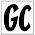### Pattern in Pascal's Triangle

Pascal's Triangle is a triangular array of binomial coefficients, and it often arises in counting problems.  In this blog post, I will present a problem that is related to Pascal's triangle and in solving it, I will derive an interesting relationship in Pascal's Triangle that you probably didn't know about.

If you randomly walk down $n$ rows of Pascal's Triangle by going down-left or down-right at each step, what is the expected value of the number that you finish on?

For example, if $n = 4$, then here are a few possible walks (that all happen to end at 4):

1.2.